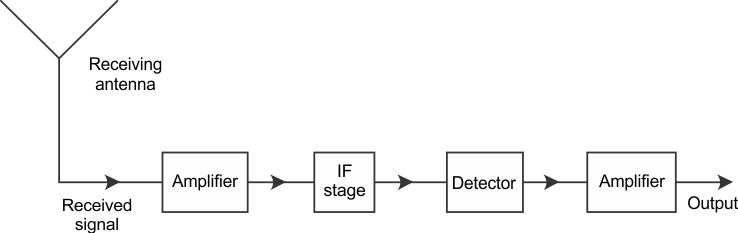# Amplitude Modulation Block Diagram

## Amplitude Modulation Block Diagram :

Amplitude Modulation Theory : In Amplitude Modulation Theory, the amplitude of a carrier signal is varied by the modulating voltage, whose frequency is invariably lower than that of the carrier. In practice, the carrier may be high-frequency (HF) while the modulation is audio. Formally, AM is defined as a system of modulation in which the amplitude of the carrier is made proportional to the instantaneous amplitude of the modulating voltage. Let the carrier voltage and the modulating voltage, νc and νm, respectively, be represented by Note that phase angle has been ignored in both expressions since it is unchanged by the Amplitude Modulation Theory process. Its inclusion here would merely complicate the proceedings, without affecting the result. However, it will certainly not be possible to ignore phase angle when we deal with frequency and phase modulation. From the definition of AM, you can see that the (maximum) amplitude Vc of the unmodulated carrier will have to be made proportional to the instantaneous modulating voltage Vm sin ωmt when the carrier is amplitude-modulated. Amplitude Modulation Block Diagram …

Generation of Amplitude Modulation : There are two types of devices in which it may be necessary to the Generation of Amplitude Modulation. The first of these, the AM transmitter, generates such high powers that its prime requirement is efficiency, so quite complex means of AM generation may be used. The other device is the (laboratory) AM generator. Here AM is produced at such a low power level that simplicity is a more important requirement than efficiency. Although the methods of generating AM described here relate to both applications, emphasis will be put on methods of generating high powers. Basic Requirements: In order to generate the AM wave of Figure 3-4b, it is necessary merely to apply the series of current pulses of Figure 3-4a to a tuned (resonant) circuit. Each pulse, if it were the only one, would initiate a damped oscillation in the tuned circuit. The oscillation would have an initial amplitude proportional to the size of the current pulse and a decay rate dependent on the time constant of the circuit. …